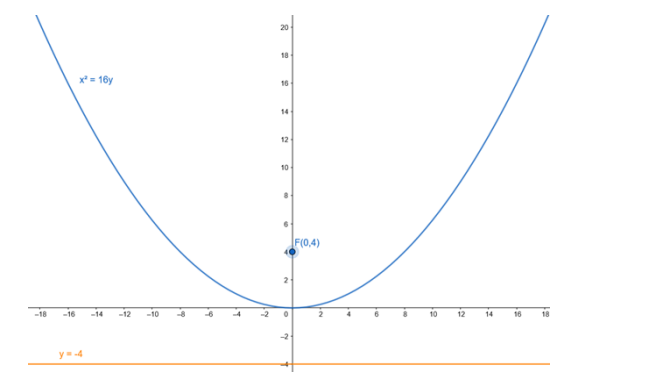# Find the coordinates of the focus and the vertex, the equations of the`
Question:

Find the coordinates of the focus and the vertex, the equations of the directrix and the axis, and length of the latus rectum of the parabola :

$x^{2}=16 y$

Solution:

Given equation : $x^{2}=16 y$

Comparing given equation with parabola having equation,

$x^{2}=4 a y$

$4 a=16$

$\cdot a=4$

Focus: $\mathrm{F}(0, \mathrm{a})=\mathrm{F}(0,4)$

Vertex : $A(0,0)=A(0,0)$

Equation of the directrix : $y+a=0$

$-y+4=0$

$\cdot y=-4$

Lenth of latusrectum : 4a = 16